# [B] Dots on the Ball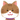halphy 自動ジャッジ 難易度: 数学 > 高校数学
2020年8月15日18:00 正解数: 10 / 解答数: 22 (正答率: 45.5%) ギブアップ不可
この問題はコンテスト「KOH Mathematical Contest #2」の問題です。

2023年3月9日10:26 [B] Dots on the Balltima_C

2021年9月3日22:17 [B] Dots on the Ball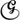Gauss

2020年8月18日0:37 [B] Dots on the Ballsapphire15

2020年8月16日10:50 [B] Dots on the Balltsukasa

2020年8月15日23:54 [B] Dots on the Ballkm299792458

2020年8月15日20:19 [B] Dots on the Ball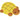takeheroaf

2020年8月15日19:58 [B] Dots on the Ballbaba

2020年8月15日19:11 [B] Dots on the Ballmochimochi

2020年8月15日19:01 [B] Dots on the Ball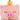BUTATA

2020年8月15日18:57 [B] Dots on the BallBUTATA

2020年8月15日18:51 [B] Dots on the BallBUTATA

2020年8月15日18:46 [B] Dots on the BallBUTATA

2020年8月15日18:41 [B] Dots on the BallBUTATA

2020年8月15日18:36 [B] Dots on the Ballbekasa001

2020年8月15日18:19 [B] Dots on the Ballrusa6111

2020年8月15日18:19 [B] Dots on the Ball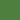green+

2020年8月15日18:16 [B] Dots on the Ballrusa6111

2020年8月15日18:15 [B] Dots on the Ballrusa6111

2020年8月15日18:12 [B] Dots on the Ball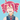nesya

2020年8月15日18:12 [B] Dots on the Ballrusa6111

2020年8月15日18:10 [B] Dots on the Ballnesya

2020年8月15日18:06 [B] Dots on the Ballnesya

### おすすめ問題

この問題を解いた人はこんな問題も解いています

#### [C] 奇妙な数列ofukufukufuku 自動ジャッジ 難易度:
3年前

#### 問題文

$$a_n=1+\sum_{m=1}^{2^n}{\rm floor}\left[\sqrt[n]{\frac{n}{\displaystyle{\sum_{k=1}^m}\; {\rm floor}\left(\cos^2\cfrac{(k-1)!+1}{k}\pi\right)}}\right]$$なお、${\rm floor}(x)$ は $x$ 以下の最大の整数を返す関数とする。このとき、$a_{20}$ を求めよ。

ただし、必要であれば以下の定理および不等式を用いても良い。

1. $n$ が素数のとき
$$\quad(n-1)!\equiv-1 \pmod n$$
2. $n\geq 1$ のとき
$$1\leq\sqrt[n]{n}<2$$

#### [E] modじゃんけん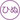hinu 自動ジャッジ 難易度:
3年前

#### 問題文

$n\;(\geq 2)$ を自然数とするとき，以下の試行を行うことを考える。

• $n$ 人が $0,1,2$ のいずれかひとつの数を無作為に選ぶ。
• 人 $i\; (i=1,2,\cdots, n)$ が選んだ数を $a_i$ とする。各人 $i$ に対して，
$$a_i\equiv\sum_{j=1}^n a_j\; ({\rm mod} \; 3)$$ならば人 $i$ は生存し，そうでないなら脱落する。この試行をmodじゃんけんと呼ぶことにする。

$n$ 人がmodじゃんけんを $1$ 回行い，全員が生存するか全員が脱落するとき，modじゃんけんの結果はあいこになると定義する。

$n$ 人がmodじゃんけんを $1$ 回行ってあいこになる確率を $p_n$ とするとき

$$p_2=\frac{\fbox{ア}}{\fbox{イ}},\; p_3=\frac{\fbox{ウ}}{\fbox{エ}},\; p_4=\frac{\fbox{オ}}{\fbox{カキ}}$$

である。$n$ を $\fbox{ク}$ で割った余りが $\fbox{ケ}$ であるとき

$$p_n=\frac{\fbox{コ}^{n}+\fbox{サ}}{\fbox{シ}^n}$$

であり，そうでないときには

$$p_n=\frac{\fbox{コ}^{n}+\fbox{ス}}{\fbox{シ}^n}$$

である。また，

$$\lim_{n\to\infty} p_n=\fbox{セ}$$

が成り立つ。

#### [A] よくある級数ofukufukufuku 自動ジャッジ 難易度:
3年前

#### 問題文

$y=\tan x \; \left(-\cfrac{\pi}{2}<x<\cfrac{\pi}{2}\right)$ の逆関数を $x=f(y)$ とする.このとき,
$$S=\sum_{n=0}^\infty f\left(\frac{1}{n^2+n+1}\right)$$を求めよ.答えは,整数ア・イを用いて
$$S=\frac{\fbox{ア}}{\fbox{イ}}\pi$$と既約分数の形でかける.

#### 解答形式

アとイをそれぞれ１行目、２行目に半角数字で入力せよ.

#### Second Numberokapin 自動ジャッジ 難易度:
3年前

### 問題文

$\sqrt {10}$ の小数第一位の値を求めよ。
ただし, $\log_{10}{2}=0.3010$ とする。

### 解答形式

#### [B] constant variableBenzenehat 自動ジャッジ 難易度:
2年前

#### 問題文

ある大きさの球から、ある直径の円柱をくりぬいた。円柱の軸は球の中心を通る。（ビーズのような形を想像してください）
この立体の体積が$36\pi$のとき、以下のうちいずれかの値が一意に定まる。

1. 円柱の底面の半径
2. 球の半径
3. 円柱の深さ1
4

#### Sandwichhalphy 自動ジャッジ 難易度:
3年前

#### 問題文

ピザが1枚ずつ乗った $N\;(\geq 2)$ 枚の皿が横一列に並んでいます．ピザにはがあり，表には具がのっていて，裏にはのっていません．はじめ，すべての皿のピザは表が上になっています．これらのピザに対して，次の操作Xを考えます．

1. 隣り合う2枚の皿に着目し，左側の皿に乗っているピザをひっくり返し，右側の皿の一番上に重ねる．ピザが複数枚乗っている場合は，ピザを重ねたまままるごとひっくり返す．
2. 左側の皿を取り除き，皿どうしのすき間を詰める．

この操作Xを$\;N-1\;$回繰り返すと，1枚の皿にピザの塔ができます．操作Xの $N-1$ 回の繰り返しをピザの調理ということにします．ピザの塔を構成するピザを，上から順に$\;P_i\; (i=1,\cdots, N)\;$とし，$P_i$ が表を上に向けているとき「表」，裏を上に向けているとき「裏」と書くことにすると，ピザの塔は「裏裏裏表」のように表すことができます．

$N=6$とします．「裏裏裏裏表表」というピザの塔ができるような調理は何通りあるか答えなさい．

#### 求面積問題3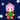Kinmokusei 自動ジャッジ 難易度:
3年前

#### 問題文

このとき、緑色部分（凹四角形）の面積を求めてください。#### 解答形式

$答えはA\sqrt{B}の形になります。(A,Bは自然数)$
$A+Bを解答してください。$
$<注意>$
$根号の中が最小となるようにしてください。$
$半角数字で解答してください。$
$例 : green area=10\sqrt{8}=20\sqrt{2}→A=20,B=2→22 と解答$

#### max漸化式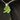masorata 自動ジャッジ 難易度:
2年前

### 問題文

$$a_1=2,\ a_2=3,\ a_{n+1} = \max_{1 \leqq k \leqq n} \{ (n-k+1)a_k \}\ (n \geqq 2)$$

で定める。$\{ a_n \}$ の一般項を求め、さらに $\log_{3}{(a_{6062})}$ の値を求めよ。

### 解答形式

$\log_{3}{(a_{6062})}$ はある自然数となるので、その値を半角数字で答えよ。

#### 求角問題2Kinmokusei 自動ジャッジ 難易度:
3年前

### 問題文

このとき、Xの角度を求めてください。### 解答形式

「度」や「°」は付けないでください。

#### 求角問題4Kinmokusei 自動ジャッジ 難易度:
3年前

#### 問題文

ただし、図中"center"で示した点は正六角形の外心です。#### 解答形式

0~360までの半角数字で、「°」や「度」をつけずに解答してください。

#### 求面積問題5Kinmokusei 自動ジャッジ 難易度:
3年前

#### 問題文

ただし、図中の青点はそれぞれの正方形の対角線の交点です。#### [D] Eigensequencehalphy 自動ジャッジ 難易度:
3年前

#### 問題文

$$a_{n+3}=3a_{n+2}-4a_{n+1}+2a_n\quad (n=1,2,\cdots)$$および
$$a_1=1, \; a_2=0, \; a_3=0$$を満たす数列 $\{a_n\}$ を考える。次の空欄 $\fbox{ア}$ 〜 $\fbox{フ}$ に当てはまる数字を答えなさい。

• 漸化式
$$a_{n+3}=3a_{n+2}-4a_{n+1}+2a_n\quad (n=1,2,\cdots)$$を満たす数列全体の集合を $V$ とする。数列 $a_n, b_n\in V$ および $c\in\mathbb{C}$ に対して，第 $n$ 項が $ca_n, a_n+b_n$ であるような数列をそれぞれ数列 $a_n$ の $c$ 倍，数列 $a_n, b_n$ の和と定義することにすると，この和とスカラー倍により $V$ は $\mathbb{C}$ 上のベクトル空間になる（確かめよ）。ここで，$V$ の元 $a_n$ は，$a_1, a_2, a_3$ を定めることで完全に決定できる。すなわち，写像 $\varphi: V \to \mathbb{C}^3$ を
$$\varphi(a_n)=\begin{pmatrix} a_1 \\ a_2 \\ a_3\end{pmatrix}$$で定めると，$\varphi$ は全単射である。しかも，$\varphi$ は線型写像だから，$\varphi$ はベクトル空間の同型になる。$V$ は $\fbox{ア}$ 次元である。また，$e_n^{(1)}, e_n^{(2)}, e_n^{(3)}\in V$ を
$$\varphi(e_n^{(1)})=\begin{pmatrix} 1 \\ 0 \\ 0\end{pmatrix},\; \varphi(e_n^{(2)})=\begin{pmatrix} 0 \\ 1 \\ 0\end{pmatrix},\; \varphi(e_n^{(3)})=\begin{pmatrix} 0 \\ 0 \\ 1\end{pmatrix}$$となるように定めると，$e_n^{(1)}, e_n^{(2)}, e_n^{(3)}$ は $V$ の基底になる。

• $V$ 上の線型変換 $L: V\to V$ を次のように定義する。$a_n\in V$ に対して，$L(a_n)$ を第 $1, 2, 3$ 項がそれぞれ $a_2, a_3, a_4$ である数列とする（$L$ が線型写像になることを確かめよ）。このとき，$L(a_n)$ の第 $n$ 項は $a_{n+\fbox{イ}}$ である。基底 $e_n^{(1)}, e_n^{(2)}, e_n^{(3)}$ のもとでの $L$ の表現行列 $L_A$ は
$$L_A=\begin{pmatrix} \fbox{ウ} & \fbox{エ} & * \\ \fbox{オ} & \fbox{カ} & \fbox{キ} \\ \fbox{ク} & \fbox{ケコ} & \fbox{サ}\end{pmatrix}$$である。

• $L_A$ の固有値を $\lambda^{(1)}, \lambda^{(2)}, \lambda^{(3)}$ とする（$\lambda^{(1)}\in\mathbb{R}, {\rm Im}(\lambda^{(2)})>0, {\rm Im}(\lambda^{(3)})<0$）。このとき
\begin{align}
\lambda^{(1)}&=\fbox{シ}\\
{\rm Re}(\lambda^{(2)})={\rm Re}(\lambda^{(3)})&=\fbox{ス}\\
{\rm Im}(\lambda^{(2)})=-{\rm Im}(\lambda^{(3)})&=\fbox{セ}
\end{align}である。

• 固有値 $\lambda^{(1)}, \lambda^{(2)}, \lambda^{(3)}$ に対応する固有ベクトルをそれぞれ $\alpha^{(1)}, \alpha^{(2)}, \alpha^{(3)}$ とする。固有ベクトルには定数倍の不定性があるが，$\alpha^{(j)}\;(j=1,2,3)$ の第 $1$ 成分が固有値 $\lambda^{(j)}$ に一致するようにとると
\begin{align}
\alpha^{(1)}=\begin{pmatrix} \lambda^{(1)} \\ \fbox{ソ} \\ * \end{pmatrix},\; \alpha^{(2)}=\begin{pmatrix} \lambda^{(2)} \\ \fbox{タ}\;i \\ * \end{pmatrix},\; \alpha^{(3)}=\begin{pmatrix} \lambda^{(3)} \\ * \\ \fbox{チツ}-\fbox{テ}\;i \end{pmatrix}
\end{align}である。

• $\varphi(\beta_n^{(1)})=\alpha^{(1)}, \;\varphi(\beta_n^{(2)})=\alpha^{(2)}, \;\varphi(\beta_n^{(3)})=\alpha^{(3)}$ となる数列 $\beta_n^{(1)}, \beta_n^{(2)}, \beta_n^{(3)}\in V$ をとる。$\beta_n^{(1)}, \beta_n^{(2)}, \beta_n^{(3)}\in V$ は $V$ の基底をなすから，$V$ の任意の元 $a_n$ はこれらの線型結合で表すことができる。例えば，$a_n\in V$ が
$$a_1=1, \; a_2=0, \; a_3=0$$を満たすとき
$$a_n=\fbox{ト}\;\beta_n^{(1)}-\frac{\beta_n^{(2)}-\beta_n^{(3)}}{\fbox{ナ}\; i}$$が成り立つ。これを変形すると
$$a_n=\fbox{ニ}-\left(\sqrt{\fbox{ヌ}}\;\right)^n\sin\left(\frac{n\pi}{\fbox{ネ}}\right)$$となる。また，$a_1,\cdots, a_{100}$ のうち $a_n$ が最大となるのは $n=\fbox{ノハ}, \fbox{ヒフ}$ のときである。ただし $\fbox{ノハ} < \fbox{ヒフ}$ とする。

※この問題では，数列とは写像 $a: \mathbb{N} \to \mathbb{C}$ のことをいう。$n\in\mathbb{N}$ に対して，$a(n)$ のことを単に $a_n$ と表記する。また，記号の濫用であるが $a$ を $\{a_n\}, a_n$とも書く。